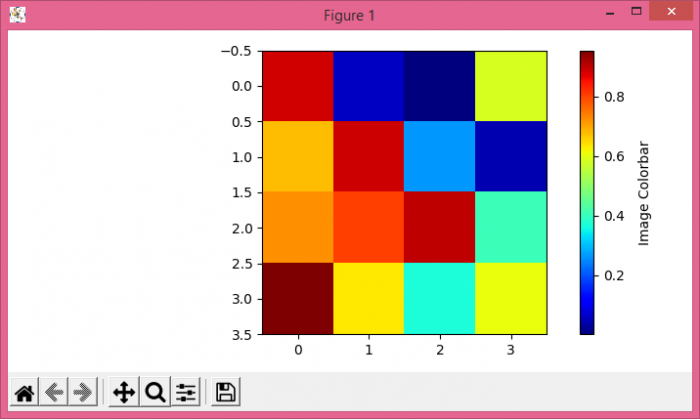# How do I adjust (offset) the colorbar title in Matplotlib?

To adjust (offset) the colorbar title in matplotlib, we can take the following steps −

• Create a random data of 4×4 dimension.

• Use imshow() method to display the data as an imgage.

• Create a colorbar for a scalar mappable instance using colorbar() method, with im mappable instance.

• Now, adjust (offset) the colorbar title in matplotlib, with labelpad=-1. You can assign different values to labelpad to see how it affects the colorbar title.

• To display the figure, use show() method.

## Example

import numpy as np
from matplotlib import pyplot as plt, cm
plt.rcParams["figure.figsize"] = [7.00, 3.50]
plt.rcParams["figure.autolayout"] = True
data = np.random.rand(4, 4)
im = plt.imshow(data, cmap=cm.jet)
cb = plt.colorbar(im)
plt.show()